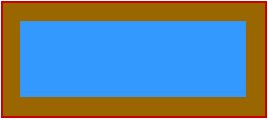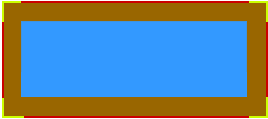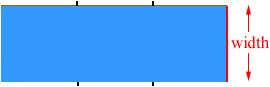Quandaries and Queries A pond is enclosed by a wooden deck that is 3 feet wide. The fence surrounding the deck is 100 feet long. If the pond is square, what are its dimensions? If the pond is rectangular and the length of the is three times its width, what are the dimensions of the pond? If the pond is circular, what is the diameter of the pond? Which pond has the most area? Dat Nguyen Hi Dat, I'll do the second part of this problem for you and then you should be able to do the others. First draw a diagram. The pool is blue, the deck is brown and the fence is red.The fence is 100 feet long. How much fence would it take if you only wanted to surround just the pool and not the deck? You could eliminate the 4 L-shaped pieces of fencing that I coloured yellow in the diagram below. Each of these pieces is 3 feet by 3 feet so you could eliminate 38 = 24 feet of fencing. Hence the perimeter of the pool is 100 - 24 = 76 feet.Now look at the pool by itself. It is 3 times as long as it is wide. If you had a length of fence that is enough for the width of the pool (I coloured it red) then, as you can see by the diagram, you would need 8 of these lengths to surround the pool. Thus perimeter = 76 feet = 8the width. Thus the width of the pool is 9.5 feet.Since the pool is 3 times as long as it is wide its length is 39.5 = 28.5 feet. Cheers, Penny Go to Math Central